Question
14.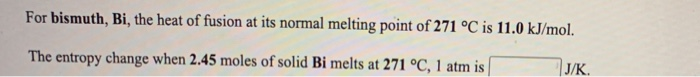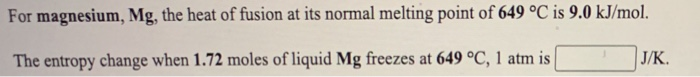We need at least 10 more requests to produce the answer.

0 / 10 have requested this problem solution

The more requests, the faster the answer.

All students who have requested the answer will be notified once they are available.

#### Earn Coins

Coins can be redeemed for fabulous gifts.

Similar Homework Help Questions
• ### The enthalpy of fusion of bismuth at its normal melting point of 271 °C is 11.0...

The enthalpy of fusion of bismuth at its normal melting point of 271 °C is 11.0 kJ mol-1. What is the entropy of fusion of bismuth at this temperature? ΔSfus =  J mol-1 K-1

• ### For benzene, C6H6, the heat of fusion at its normal melting point of 6 °C is...

For benzene, C6H6, the heat of fusion at its normal melting point of 6 °C is 10.0 kJ/mol. The entropy change when 1.86 moles of liquid C6H6 freezes at 6 °C, 1 atm is ? J/K.

• ### For ethanol, C2H5OH, the heat of fusion at its normal melting point of -115 °C is...

For ethanol, C2H5OH, the heat of fusion at its normal melting point of -115 °C is 5.0 kJ/mol. The entropy change when 1.84 moles of solid C2H5OH melts at -115 °C, 1 atm is J/K.

• ### For copper, Cu, the heat of fusion at its normal melting point of 1083 °C is...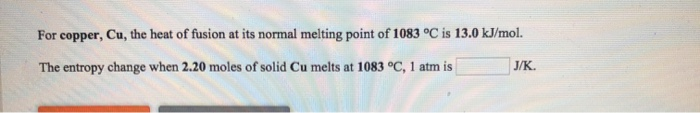For copper, Cu, the heat of fusion at its normal melting point of 1083 °C is 13.0 kJ/mol. The entropy change when 2.20 moles of solid Cu melts at 1083 °C, 1 atm is J/K

• ### For bismuth, Bi, the heat of vaporization at its normal boiling point of 1627 °C is...

For bismuth, Bi, the heat of vaporization at its normal boiling point of 1627 °C is 172.0 kJ/mol. The entropy change when 2.11 moles of Bi vapor condenses at 1627 °C, 1 atm is J/K.

• ### The molar enthalpy of fusion of solid bismuth is 11.0 kJ mol-1, and the molar entropy...

The molar enthalpy of fusion of solid bismuth is 11.0 kJ mol-1, and the molar entropy of fusion is 20.2 J K-1 mol-1. (a) Calculate the Gibbs free energy change for the melting of 1.00 mol of bismuth at 575 K. kJ (b) Calculate the Gibbs free energy change for the conversion of 5.12 mol of solid bismuth to liquid bismuth at 575 K. kJ (c) Will bismuth melt spontaneously at 575 K? (d) At what temperature are solid and...

• ### The enthalpy of fusion of cadmium at its normal melting point of 321 °C is 6.11...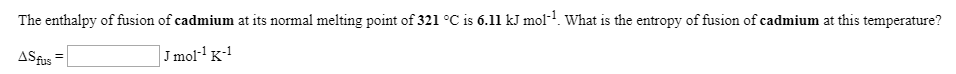The enthalpy of fusion of cadmium at its normal melting point of 321 °C is 6.11 kJ mol? What is the entropy of fusion of cadmium at this temperature? ASfus = J mol-K-1 The molar enthalpy of fusion of solid cadmium is 6.11 kJ mol-1, and the molar entropy of fusion is 10.3 JK+mol-1. (a) Calculate the Gibbs free energy change for the melting of 1.00 mol of cadmium at 622 K. (b) Calculate the Gibbs free energy change for...

• ### 6. The melting point of CaTiSiOs is 1400°C and the heat of fusion at the normal melting point is 123 700 J mol-1. Calculate the heat of fusion at 1300°C. Cnsolid = 177.4 + 23.2 x 10', 40.3 x...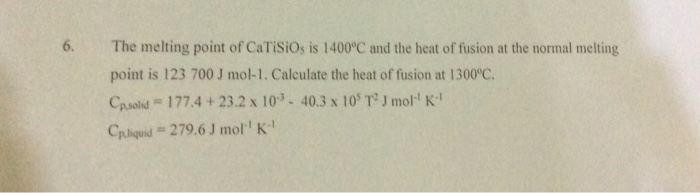6. The melting point of CaTiSiOs is 1400°C and the heat of fusion at the normal melting point is 123 700 J mol-1. Calculate the heat of fusion at 1300°C. Cnsolid = 177.4 + 23.2 x 10', 40.3 x 105 T2 J mor' K" Cphquid # 279.6 J mol'' K" 6. The melting point of CaTiSiOs is 1400°C and the heat of fusion at the normal melting point is 123 700 J mol-1. Calculate the heat of fusion at 1300°C....

• ### The enthalpy of fusion of manganese at its normal melting point of 1244 ? is 14.6...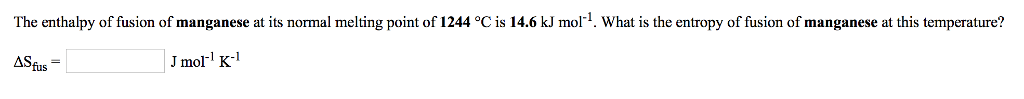The enthalpy of fusion of manganese at its normal melting point of 1244 ? is 14.6 kJ mol-1 What is the entropy of fusion of manganese at this temperature? ASfus Jmol K

• ### For silicon, Si, the heat of vaporization at its normal boiling point of 2355 °C is...

For silicon, Si, the heat of vaporization at its normal boiling point of 2355 °C is 297.1 kJ/mol. The entropy change when 2.26 moles of Si vapor condenses at 2355 °C, 1 atm is J/K.